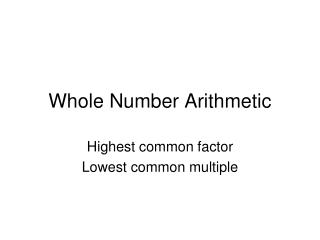Download PresentationWhole Number Arithmetic

# Whole Number Arithmetic - PowerPoint PPT Presentation

Whole Number Arithmetic. Highest common factor Lowest common multiple. H.C.F (highest common factor). { factors of 8 } = { 1, 2, 4, 8 } { factors of 12 } = { 1, 2, 3, 4, 6, 12 } The H.C.F. is 4. L.C.M (lowest common multiple). { multiples of 8 } = { 8, 16, 24, 32 … }I am the owner, or an agent authorized to act on behalf of the owner, of the copyrighted work described.
Download Presentation## Whole Number Arithmetic

Download Policy: Content on the Website is provided to you AS IS for your information and personal use and may not be sold / licensed / shared on other websites without getting consent from its author.While downloading, if for some reason you are not able to download a presentation, the publisher may have deleted the file from their server.

- - - - - - - - - - - - - - - - - - - - - - - - - - E N D - - - - - - - - - - - - - - - - - - - - - - - - - -
Presentation Transcript
1. Whole Number Arithmetic Highest common factor Lowest common multiple

2. H.C.F (highest common factor) • { factors of 8 } = { 1, 2, 4, 8 } • { factors of 12 } = { 1, 2, 3, 4, 6, 12 } • The H.C.F. is 4

3. L.C.M (lowest common multiple) • { multiples of 8 } = { 8, 16, 24, 32… } • { multiples of 12 } = {12, 24, 36… } • The L.C.M. is 24

4. H.C.F 2 L.C.M 6 2 and 6

5. H.C.F 2 L.C.M 10 2 and 10

6. H.C.F 3 L.C.M 6 3 and 6

7. H.C.F 3 L.C.M 12 3 and 12

8. H.C.F 4 L.C.M 12 4 and 12

9. H.C.F 5 L.C.M 10 5 and 10

10. H.C.F 2 L.C.M 12 4 and 6

11. H.C.F 2 L.C.M 24 6 and 8

12. H.C.F 3 L.C.M 18 6 and 9

13. H.C.F 3 L.C.M 36 9 and 12

14. H.C.F 2 L.C.M 20 4 and 10

15. H.C.F 2 L.C.M 30 6 and 10

16. H.C.F 2 L.C.M 8 2, 4 and 8

17. H.C.F 2 L.C.M 24 4, 6 and 8

18. H.C.F 3 L.C.M 12 3, 6 and 12

19. H.C.F 3 L.C.M 36 6, 9 and 12

20. 5 is a factor of 10. 5 is a multiple of 10. 10 is a factor of 5. 10 is a multiple of 5. 7 is a factor of 7. 7 is a multiple of 7. 7 is a prime number. 9 is a prime number. 3 is a prime factor of 12. True False False True True True True False true Exercise 7 - Oral examplesTrue or False

21. Set Notation • If x is an element (or member) of a set A then we write • If x is not an element of a set A then we write . • e.g. if • A = { factors of 10 } = { 1, 2, 5, 10 } • Then , • Whereas .

22. If all the elements of A are also elements of B then we say that A is a subset of B and write • e.g. If A = {prime factors of 10} = {2, 5} • and B = {factors of 10} = {1, 2, 5, 10} then

23. 2 _____ { 1, 2, 3 } { 2 } ____ { 1, 2, 3 } 7 ______ { 4, 5, 6 } { 7 }____ { 4, 5, 6 } 3 _____ { 2, 3, 4 } 8 ______ { 5, 6, 7 } { 3 } ____ { 2, 3, 4 } { 8 } ____ { 5, 6, 7 } Exercise 7Choose the correct symbol

24. Exercise 7 • 5 ______ { factors of 5 } • 5 _____ { multiples of 5 } • 4 _______ { factors of 8 } • 4 ______ { multiples of 8 } • 6 _________ { factors of 3 } • 6 __________ { multiples of 3 } • { 5 }______ { factors of 5 } • { 5 } _______ { multiples of 5 }

25. Exercise 7 • { 4 } ____ { factors of 8 } • { 4 } _____ { multiples of 8 } • { 6 } _____ { factors of 3 } • { 6 } _____ { multiples of 3 } • 17 ______ { prime numbers } • { 17 } ____ { prime numbers } • 27 _____ { prime numbers } • { 27 } ____ { prime numbers }

26. Exercise 7 • 1 ____ { prime factors of 3 } • 2 ____ { prime factors of 6 } • { 3 }____ { prime factors of 9 } • { 4 } _____{ prime factors of 12}